Dark Matter is a form of matter which is thought to account for approximately 85% of the matter in the universe and the remaining is made up visible or baryonic matter. Its presence is implied in a variety of astrophysical observations, including the gravitational affects has on the orbits of stars in galaxies which cannot be explained by accepted theories of gravity unless more matter is present than can be seen. The reason it is called dark is because it does not appear to interact with the electromagnetic field, which means it does not absorb, reflect or emit electromagnetic radiation, which is why it is difficult to detect.However, we disagree that it cannot be explained by accepted theories of gravity because Einstein defined gravity in terms of the “depth” of a gravity well or distortion in the “surface” of space-time caused by the energy density of an environment and NOT on existence of visible or baryonic matter. This means the energy of electromagnetic fields, photons and all other forms of energy along with that associated with visible matter must be taken consideration when determining the energy density of space and therefore its gravitational potential.

This suggests the reason it does not appear to interact with an electromagnetic field is because a large part it MAY BE made up of an electromagnetic field.

The observation electromagnetic energy prevents the visible matter in stars from collapsing to a black hole or falling to the button of its gravity well supports this conclusion because it tells us its gravitational potential MUST BE oppositely directed with respect to that of visible matter.

Some might say, if that were true it should have the same effect on the orbits of planets as it does on stars in galaxies. However, the reason it DOES NOT is because the offset it creates would be affect the gravitational field of the entire solar system.  Therefore, objects that are gravitationally bound to a star would only experience the gravitational potential of its visible matter.

One can understand why by using an analogy of a jar containing water and oil where the water represents gravitational potential of electromagnetic energy while the oil represents that of visible matter. The water prevents the oil from sinking to the bottom because its “directional energy” is opposite or is more buoyant than the water. This would be analogous to how electromagnetic energy prevents the visible matter in stars from sinking to the bottom of a stars gravity well.

However, as was mentioned earlier Einstein defined gravity in terms of the “depth” of a gravity well or distortion in the “surface” of space-time caused by the energy density of an environment NOT on existence of visible of baryonic matter.

Therefore, to determine the TOTAL gravitational potential or depth of the gravity well of a solar system one must ADD the energy density associated with electromagnetic energy to that of its visible matter.

Yet, to define the gravitational potential on objects which ARE GRAVITATIONALLY bound to a star one would HAVE TO use only that contributed by the visible matter because, as mentioned earlier a solar systems gravity well is offset by the electromagnetic energy. Therefore, any objects gravitational bound to a star would only be affected by the gravitational potential of the visible matter.

This would be analogous how one inside the jar, mentioned above would measure the height of the oil with respect to the water line.

Similarly, a planet inside a solar system would measure the gravitational potential it would experience from “height” associated with the visible matter with respect to the “height” of the gravitational potential of its electromagnetic energy.

One can also use the example of the jar mentioned earlier to understand why galaxies in galactic clusters and stars orbiting in them are affected by BOTH the gravitational potential of electromagnetic energy and visible matter.

For example, one outside the jar would add the height of the oil to the water to get its total height

Similarly, galaxies in galactic clusters and stars that are not gravitational bound to a solar system would experience the gravitational potential contributed by both the visible matter electromagnetic energy for the same reason that one who was outside the jar mentioned earlier would measure the height above its bottom in by adding the height of the water to the oil.

This means according to Einstein the total gravitation potential of the universe must be at least TWICE that contributed by the visible matter of a healthy star because it is in equilibrium with oppositely directed gravitational energy he associated with its electromagnetic energy.

He also tells us ANY FORM of energy that COUNTERACTS that of the gravitational potential of visible matter must also be consider a component of the Dark Matter. For example, the orbital energy of the stars in a galactic would have to be included because it also adds to the energy density of the space they occupy. In other words, not only do you have to add the energy density contributed by electromagnetic energy to that of the visible matter in stars but you must also add their orbital energy to determine to determine their total gravitational potential of a galaxy.  Additionally, the fact that galaxies are gravitational bound in galactic clusters means you must also consider the energy density contributed by their rotational energy to the Dark mater component of the universe.

Additionally, the OBSERVATION that electromagnetic energy offsets the gravitational potential of the visible matter tells us that it must contribute AT LEAST an equal amount to universe total. The remaining Dark matter could be provided by the energy density contributed by dust, helium atoms, black holes along their orbital energy.

It should be remembered; Einstein defined the depth of a gravity well in space in terms of the ABSOLUTE value of its energy density. Therefore, to determine the total gravitational potential of both Dark and visible matter one must include all forms of energy to determine their value.

There are two ways science attempts to explain and define the behavior of our universe. The first is Quantum mechanics or the branch of physics defines its evolution in terms of the probabilities associated with the wave function. The other is the deterministic universe of Einstein which defines it in terms of a physical interaction between space and time.

Specifically, Einstein defined the position a particle in terms of where an increase in the energy density in space associated with its mass is located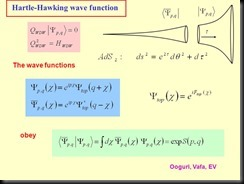While quantum mechanics uses the mathematical interpretation of the wave function to define the most probable position of a particle when observed.

Since we all live in the same world you would expect the probabilistic approach of quantum mechanics to be compatible with the deterministic one of Einstein. Unfortunately, they define two different worlds which appear to be incompatible. One defines existence in terms of the probabilities while the other defines it in terms of the deterministic of properties of space and time.

However, even though those probabilities appears to be incompatible with Relativity’s determinism they can be explained in terms of a physical interaction between space and time.

For example, when we role dice in a casino most of us realize the probability of a six appearing is related to or caused by its physical interaction with properties of the table in the casino where it is rolled. Putting it another way what defines the fact that six appears is NOT the probability of getting one but the interaction of the dice with the table and the casino it occupies.

Therefore, to integrate the probabilistic interpretation of the wave function in terms of the deterministic properties of space and time one must show how and why an interaction between them is responsible for the position of a particle when observed .

One way of doing this is to use the fact that evolution in both a quantum and space-time environments are controlled by a wave.

For example, Relativity defines evolution of space-time in terms of the energy propagated by electromagnetic wave while Quantum Mechanics defines it in terms of the mathematical evolution of the wave function.

This suggests the wave function that governs the evolution of a quantum environment may be a mathematical representation of an electromagnetic wave that governs evolution in the world of Relativity.  If true one should be able to derive the probabilistic predictions of quantum mechanics in terms of the deterministic properties of an electromagnetic wave in space-time.

For example, the science of wave mechanics along with the fact Relatively tells us electromagnetic moves continuously through space-time unless it is prevented from doing so by someone observing or something interacting with it. This would result in its energy being confined to three-dimensional space. The science of wave mechanic also tells us the three-dimensional “walls” of this confinement will result in its energy being reflected back on itself thereby creating a resonant or standing wave in three-dimensional space. This would cause its wave energy to COLLAPSE and concentrated at the point in space were a particle would be found. Additionally, wave mechanics also tells us the energy of a resonant system, such as a standing wave can only take on the discrete or quantized values associated with its fundamental or a harmonic of its fundamental frequency. This means a particle would occupy an extended volume of space defined by the wavelength of its standing wave.

Putting it another way what defines the fact that a particle appears where it does is NOT determined by the probabilities associated with the wave function but the deterministic interaction of an electromagnetic wave with the physical properties of space-time

However, the probabilistic interpretation of the wave function is required because Quantum mechanics defines the position of a particle in terms of mathematical point in space which it randomly defines respect to its center. Therefore, the randomness of where that point is with respect to a particle’s center will result in its position, when observed to be randomly distributed in space. This means one must define where it appears in terms of probabilities to average the deviations that are caused by the random placement of that point.

This shows why Relativity is indeterminant on a quantum scale while also being deterministic on a macroscopic level because those deviations would be averaged out by the large number of particles in objects like the moon and planets

It shows how one can derive the “Reality” of probabilistic world of quantum mechanics in terms of the deterministic one of space-time by assuming the wavefunciton is a mathematical representation of an electromagnetic wave.

The Big Bang Theory is the leading explanation about how the universe began. At its simplest, it says the universe as we know it started with a singularity, then inflated over the next 13.8 billion years to the cosmos that we know today.

Because current instruments don’t allow astronomers to peer back at the universe’s birth, much of what we understand about the it comes from mathematical formulas and models. Astronomers can, however, see the “echo” of the expansion through a phenomenon known as the Cosmic Background Radiation.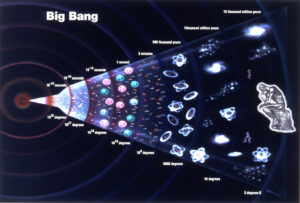The idea the universe was smaller in the beginning was supported by Edwin Hubble observation in1929 that it was expanding.

Later, a few physicists led by George Gamow a proponent of the Big Bang Model showed an expanding universe meant that it might have had its beginning in singularity or a very hot infinitely dense environment, which then expanded to generate the one we live in today.

They were able to show only radiation emitted approximately 300,000 years after the beginnings of the expansion should be visible today because before that time the universe was so hot that protons and electrons existed only as free ions making the universe opaque to radiation.

Additionally, they predicted this Cosmic Background Radiation would have cooled from several thousand degrees Kelvin back when it was created to 2.7 today due to the expansion of the universe.  Many thought its discovery 1965 by Penzias and Wilson provided its verification

However, there was a problem with assuming the universe begin that way because an infinitely dense environment must have been, by definition homogeneous. Therefore, if the universe was homogeneous when it began it should still be.

But the existence of galactic clusters and the variations in the intensity of the Cosmic Background Radiation discovered by European Space Agency’s Planck space observatory showed the universe is not and therefore, was not homogeneous either now or at the time when it was emitted.

Many proponents of the big bang model assume that these “anisotropy” in the universe are caused by quantum fluctuations in the energy density of space. They define quantum fluctuations as a temporary change in the energy of space caused by the uncertainty principle.

However, there are CATASTROPHIC THEORETICAL errors in both assuming our universe originated from a singularity and the  affects quantum fluctuations in the energy density of space would have on the evolution of the universe.

Einstein and observations of black holes tell us time moves slower as the energy density increases and will eventually stop if it becomes great enough. Additionally, Schwarzschild was able to use Einstein’s math to calculate the radius of a black hole where the energy density would be great enough to stop time which is LARGER than that of a singularity.

This tells us because expansion CANNOT occur in an environment where time has stopped there is a minimum radius the total energy content of the universe can occupy for time to move forward which IS larger than a singularity.

In other words, if the proponent of the big bang model had considered the effect energy density has on time, they would have realized that the universe could not have originated from a singularity.

Some may say that the energy density of expanding universe would not affect the rate at which time passes but they would be WRONG because Einstein’s tells us it would be related ONLY to its differential energy density. In other words, he tells us the rate at which time slows and where it would stop and prevent further expansion would be determined by the differential energy density between the center of its expansion and its outer edge.  Therefore, similar to a black hole the universe would have an “event horizon” which would define its minimum volume before which no expansion could occur.

However, there is a similar error behind the assumption that quantum fluctuations are responsible for “anisotropy” in Cosmic Background Radiation because their energy density by definition would great enough to cause time to stop.  Therefore, quantum fluctuations could not affect the evolution of the universe or be responsible for “anisotropy” in Cosmic Background Radiation because as was just mentioned evolution cannot occur in an environment where time has stopped.

Some might disagree because they say the energy in a singularity and that contained in a quantum fluctuation would be powerful enough to overcome the stopping of time predicted by Einstein mathematics.  However, they would be WONG again because Einstein tells that when the energy density reaches a certain level time will stop.  It does not say that an increase beyond that point will allow time to move again.

As was mentioned earlier, current instruments don’t allow astronomers to peer back at the universe’s birth, much of what we understand about its origin comes from theory and mathematical formulas.

However, we may be able to define the origin of the present universe in terms of its observable properties.

We still have not been able to determine if the universe will continue to expand indefinitely or if it will eventually collapse in on itself. However, if one assumes it does, one could develop a mathematically model which would allow for determining when the heat generated by its collapse would it cause it to re-expand.  If it was found it was great enough to cause protons and electrons to exist only as free ions before the radiation pressure caused it to enter an expansion phase then another round of the Cosmic Background Radiation would be created.

This would also give one the ability to determine if the anisotropy in it COULD be the result of irregularities in its collapse based on observation of the irregularities that exist today

Putting it another way we could define the origin of the present universe and anisotropy” in Cosmic Background Radiation in terms of real time observations of the present universe which would be consistent with the theoretical predictions of Einstein.

The science of Astrophysics is base almost exclusively on observations.  Therefore, the question they must ask themselves is “If we have two models for the origin of the universe that predict the same outcome which one should we assume is correct?”  The one that make define its origins based on the observable properties of our present universe or one that defines it in terms of the unobservable properties of a singularity.

Copyright Jeffery  O’Callaghan Nov. 2020

 The Road to Unifying QM with Relativity part 1 2007 thru 2010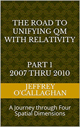Ebook \$8.00Paper Back \$15.00 The Road to Unifying QM with Relativity part 2 2011 thru 2014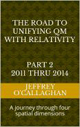Ebook \$8.00Paper Back \$16.00 The Road to Unifying QM with Relativity part 3 2015 thru 2020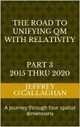Ebook \$8.00Paper Back \$18.00

Quantum electrodynamics (QED) is the relativistic quantum field theory of electrodynamics. Its a theoretical framework which combines classical field theory , special relativity and the quantum mechanical properties of particles as excited states of quantum fields , which it assumes are more fundamental than the particles. However, it has not been able to derive gravity as defined in the General Theory of Relativity into that framework.

In S pecial Relativity the exchange energy between particles is derived terms of electromagnetic waves moving though the continuous field properties of space-time. While the General Theory of Relativity derives gravity in terms of distortion or curvature in the “surface” of that field created by an increase in the local energy density.In Schrödinger’s version of QFT, the wave function defines the mathematical evolution of an ISOLATED quantum system. That along with SUPERPOSITION principle of quantum mechanics allows one to add of all possible outcomes defined by the wave function together to derive the quantum equivalent of the classical and the space-time fields of Relativity.

Putting it another way both QED and Special Relativity derive the evolution of the field properties of their environments in terms of waves. Relativity defines it in terms of the exchange of energy between objects in terms of an electromagnetic wave moving thought the field properties of space-time while QED mathematically defines it in terms of an interaction of the wave function with the field properties of all possible outcomes.

This suggests that the wave function that defines the evolution of field properties of QED may be a MATHEMATICAL representation of an electromagnetic wave that defines it in space-time.

As was mentioned earlier the General Theory of Relativity derives the “evolution” of a gravitational field in terms of distortion or curvature in the “surface” of a space-time field created by an increase in its local energy density while QED derive it in terms of how an interaction of the wave function with all possible outcomes would cause the creation of a particle and therefore an increase the energy density of space.

This suggests one way to integrate gravity into QED may be to define how and why the interaction of the field properties of all possible outcomes with the wave function causes a QUANTIZED increase its energy density.

However, one can show Relativity also define quantum gravity by showing why an interaction of an electromagnetic wave with the field properties of space-time also results in a QUANTIZED increase in the energy density of space.

In other words, one can show gravitational forces may not be quantized but their causality MAY be.

One way of accomplish this would be to use the science of wave mechanics and the fact Relatively tells us the energy of an electromagnetic wave energy would move continuously through space-time unless it is prevented from by moving through time by someone observing or something interacting with it. This would result in its energy being confined to three-dimensional space. The science of wave mechanics also tells us the three-dimensional “walls” of this confinement will result in its energy being reflected back on itself thereby creating a resonant or standing wave in three-dimensional space. This would cause its wave energy to be concentrated at the point in space where a particle would be found thereby increasing it energy density. Additionally, wave mechanics also tells us the energy of a resonant system, such as a standing wave which this confinement would create can only take on the discrete or quantized values associated with its fundamental or a harmonic of its fundamental frequency.

Putting it another way it defines quantum gravity in terms of how and why an electromagnetic wave interacts with field properties of space-time to cause a QUANTIZED increase in energy density and therefore the gravitational potential in a specific region of space.

However, to derive quantum gravity in terms of QED one must show how the interaction of the wave function with the field properties of ALL possible outcomes would result in quantum increase in the energy density of that field.

One way of doing this would be to compare the mathematical oscillations associated with the wave function in the field properties of all possible outcomes to ball oscillating on a rubber diaphragm. Observations of that diaphragm tell us those vibrations will be disturbed over its surface while its magnitude would decrease as one moves away from the focal point of the oscillations.

Similarly, if the assumption that wave function is a mathematical representation of vibrations or oscillations in the field properties of ALL possible outcomes, is correct these oscillations would be distributed the entire field while the magnitude of those oscillations would be greatest at their focal point and decreases as one moves away from it. Putting it another way, the PROBABILITY of QUANTUM increase in its energy density in space due to a particle being there would be related its distance from the focal point of those oscillations.

In other words, it is possible to understand why gravity is quantized in terms of both quantum mechanics and Relativity by assuming it is the result of interaction of a wave moving through their respective fields. For example, as was shown above when an electromagnetic wave interacts with the field properties of space-time it would create a quantized increase in the energy density of space. While, as was also shown above when the mathematical properties of the wave function interacts with the probably field associated QED it creates a particle or quantized increase in the energy density of space would be responsible for gravity.

Recently observations have suggested a force called Dark Energy is needed to account for the fact the expansion of the universe is accelerating. However. that may be an illusion created by the effect gravity has on time.

Einstein told us and it has been observed the rate at which time is perceived to move is slower in all environments where the gravitational potential is greater with respect where it is being observed. This means the further we look back in time, where universe gravitational potential of the more densely pack matter was greater the estimate of its rate of expansion would be less than it actually was if those effects were not taken into consideration. In other words, if one had not considered the Relativistic slowing of time caused by its gravitational potential that rate would be faster in the past than the present value suggests.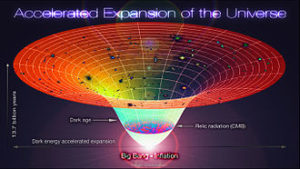However, we also know the gravitational potential has a slowing effect on the universe’s expansion and because that potential decreases as its volume increase, its expansion rate also decreases. In other words, as the universe expands the slowing effect of gravity has on it decreases.

Putting it another way, the rate of universe’s expansion would to appear to occur more rapidly than it actually did from the perspective of present due to the effect gravity has on time while its actual rate would decline due to its gravitational potential as the universe expands.

Therefore, to determine its actual rate of expansion at each point in its history one must not only take into account the gravitational effect on time that would make it appear that it was slower that it actual was but one must also consider how gravity actually causes its expansion rate to decrease.

Yet, because of the non-linear effects between the slowing of time created by universe’s gravitational potential with respect to effects it has on its rate of expansion there will be a point in its history where one will APPEAR to overtake the other.

IN OTHER WORDS, IT IS POSSIBLE THE OBSERVATIONS SUGGESTING THE UNIVERSE EXPANSION IS ACCELERATING MAY BE THE RESULT OF THE FACT THE GRAVITATIONAL POTENTIAL OF THE UNIVERSE CAUSES TIME TO APPEAR MOVE SLOWER IN THE PAST THAN IT ACTUALLY DID.

This gives us a way of validating if the gravitational potential and the slowing effect it has on time is what gives us the IMPRESSION the expansion of the universe is accelerating because it has been observed that about 4 billion years ago the universe’s expansion appears to have change from decelerating to an accelerated phase.

This because one can calculate its actual expansion rate by determining how much time would have appeared to move slower due to the differential gravitational potential between the past and present and use that value to determine its actual rate. If it was found that about 4 billion years ago the effect the slowing of time on our perception of its past expansion rate is less than slowing effect gravity would have on, it would appear as if that rate was accelerating even though it was still decelerating.

Some may say the slowing of time slowing would not affect the timing of the expansion because it is also expanding. However, Einstein define the time dilation only in terms of the affects a differential gravitational potential has on it therefore an expanding universe would not affect it. Some may also say that because the universe is expanding the gravitational potential is expanding and weakening at the same rate therefore when we look back the effects it will have on the timing of its expansion will cancel. However, Einstein tells us the timing of events that cause the universe to expand is locked in the past along with its gravitational potential at the time the expansion took place. Therefore, one must take into account the differential gravitational potential between the past and present universe when defining its expansion.

Some have also suggested Relativistic properties space have already been accounted for in the Friedman model that was used in part by scientist to define the accelerated expansion of the universe. However, that is NOT the case because when someone in the past measures the rate of change in the universe’s expansion rate he would NOT need to use it because his entire spatial slice of the universe would be at the same gravitational potential. However this would NOT be the case for someone looking at that spatial slice from the future. He would have to use it because due to the expansion of the universe a differential gravitational potential would have developed between the past and the present. But as the universe expands the rate of change of its expansion will slow because the gravitational density of the universe has decreased while at the same time the relativistic effects defined by Friedman model will also decreased because of the decrease in gravitation density between the past and present. Yet the relativistic properties of space tell us from the perspective of the present the rate of the universe’s expansion is moving faster than it actually is from the perspective of someone who is present at the time when that expansion was taking place. In other words, Friedman equation defines how the rate of the rate of universe’s expansion changes due to a differential gravitational density between a past and present but it does not define the actual rate of from the perspective of someone who was measuring it from his spatial slice. Therefore, to define the ACTUAL rate at which the expansion of the universe is changing from one point to the next one must determine how the change in gravitational densely effects it from the perspective of an observer at each point in its history and NOT with respect to observer who is looking back at it from the present as the Friedman equation does.

Copyright Jeffrey O’Callaghan Nov. 2020

Please visit our Facebook group The Road to unification of Quantum and Relativistic theories if you would like to comment or contribute to our project

 The Road to Unifying QM with Relativity part 1 2007 thru 2010Ebook \$8.00Paper Back \$15.00 The Road to Unifying QM with Relativity part 2 2011 thru 2014Ebook \$8.00Paper Back \$16.00 The Road to Unifying QM with Relativity part 3 2015 thru 2020Ebook \$8.00Paper Back \$18.00

« Previous Articles    Next Articles »
Unifying Quantum and Relativistic Theories is based on WordPress platform.